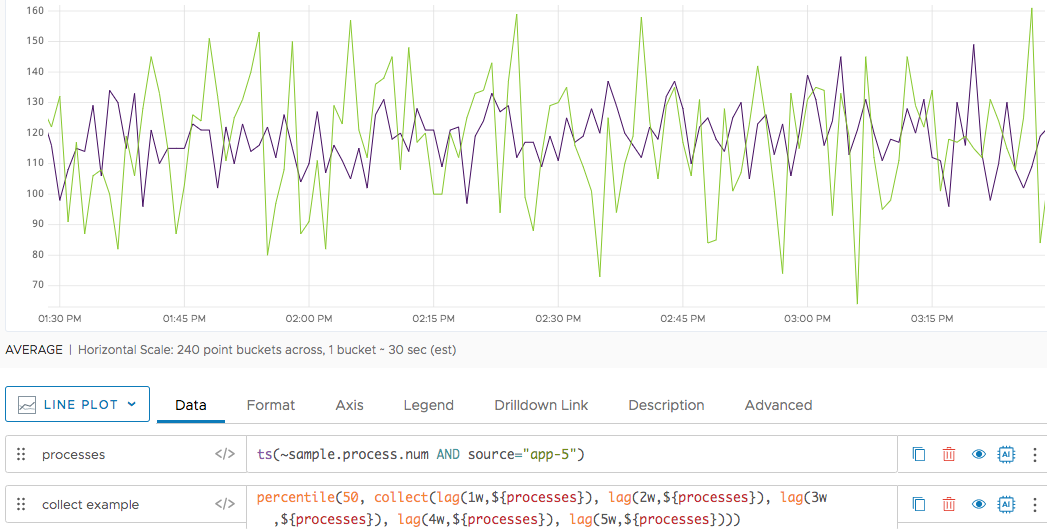Reference to the collect() function

## Summary

``````collect(<expression1>, <expression2> [, <expression3>, ...])
``````

Returns a ts() expression that is the combination of two or more ts() expressions.

## Parameters

ParameterDescription
expression A ts() expression that you want to include in the collection.

## Description

The `collect()` function returns a ts() expression that is the combination of two or more ts() expressions.

The returned expression includes a synthetic `collect_<number>` point tag, where `<number>` is the number of input expressions.

The `collect()` function is just a convenience function that combines multiple expressions into a single expression. It does not operate on the time series described by the input expressions (for example, it does not create a single series from them).

## Example

Assume you want the mean for the number of processes. You could calculate it explicitly:

``````(
lag(1w,\${processes})+
lag(2w,\${processes})+
lag(3w,\${processes})+
lag(4w,\${processes})+
lag(5w,\${processes})
) / 5
``````

The problem is, if there’s a holiday or other anomaly during one of those lags, the data are affected and the mean doesn’t tell you the complete story.

With `collect()` you can do a similar query but get the median instead of the mean. The median filters out anomalies and holidays:

``````percentile(50,collect(
lag(1w,\${processes}),
lag(2w,\${processes}),
lag(3w,\${processes}),
lag(4w,\${processes}),
lag(5w,\${processes})
))
``````

The following screen shot shows the second query, with the anomalies filtered out because we can use (`percentile(50...)`) to get the median instead of the mean.